Study Materials: ncert solutions

Our ncert solutions for Solutions 7. Integrals - Exercise 7.7 | Class 12 Mathematics-II - Toppers Study is the best material for English Medium students cbse board and other state boards students.

Solutions ⇒ Class 12th ⇒ Mathematics-II ⇒ 7. Integrals

# Solutions 7. Integrals - Exercise 7.7 | Class 12 Mathematics-II - Toppers Study

Topper Study classes prepares ncert solutions on practical base problems and comes out with the best result that helps the students and teachers as well as tutors and so many ecademic coaching classes that they need in practical life. Our ncert solutions for Solutions 7. Integrals - Exercise 7.7 | Class 12 Mathematics-II - Toppers Study is the best material for English Medium students cbse board and other state boards students.

## Solutions 7. Integrals - Exercise 7.7 | Class 12 Mathematics-II - Toppers Study

CBSE board students who preparing for class 12 ncert solutions maths and Mathematics-II solved exercise chapter 7. Integrals available and this helps in upcoming exams 2023-2024.

### You can Find Mathematics-II solution Class 12 Chapter 7. Integrals

• All Chapter review quick revision notes for chapter 7. Integrals Class 12
• NCERT Solutions And Textual questions Answers Class 12 Mathematics-II
• Extra NCERT Book questions Answers Class 12 Mathematics-II
• Importatnt key points with additional Assignment and questions bank solved.

Chapter 7 Mathematics-II class 12

### Exercise 7.7 class 12 Mathematics-II Chapter 7. Integrals

• Solutions 7. Integrals - Exercise 7.7 | Class 12 Mathematics-II - Toppers Study
• Class 12 Ncert Solutions
• Solution Chapter 7. Integrals Class 12
• Solutions Class 12
• Chapter 7. Integrals Exercise 7.7 Class 12

## Solutions 7. Integrals - Exercise 7.7 | Class 12 Mathematics-II - Toppers Study

Exercise 7.7

Integrate the functions in Exercises 1 to 9.

Ques.1.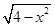Ans.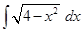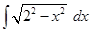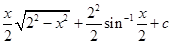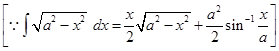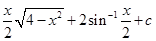Ques.2.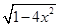Ans.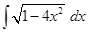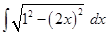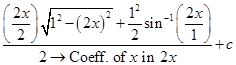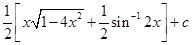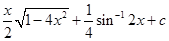Ques.3.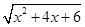Ans.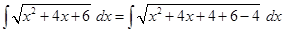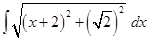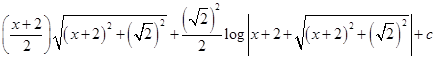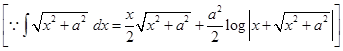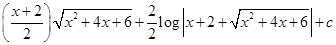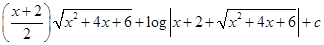Ques.4.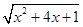Ans.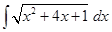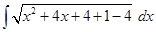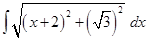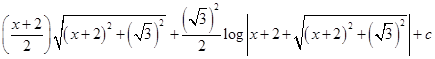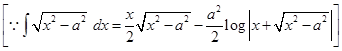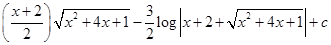Ques.5.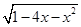Ans.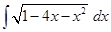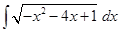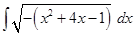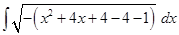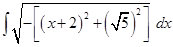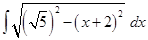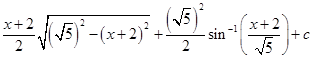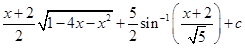Ques.6.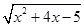Ans.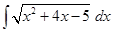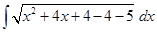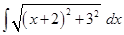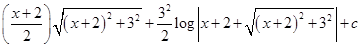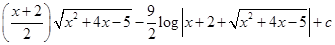Ques.7.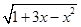Ans.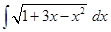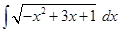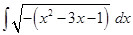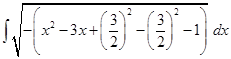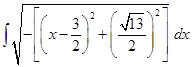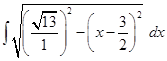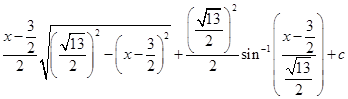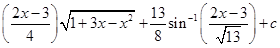Ques.8.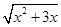Ans.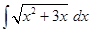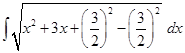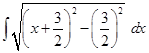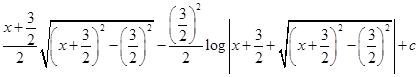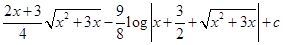Ques.9.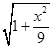Ans.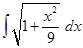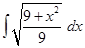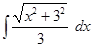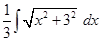=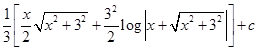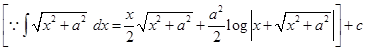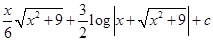Choose the correct answer in Exercise 10 to 11.

Ques.10.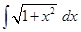is equal to:

(A)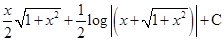(B)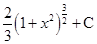(C)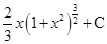(D)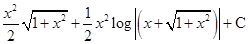Ans.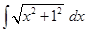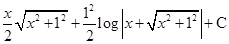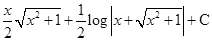hance, option (A) is correct.

Ques.11.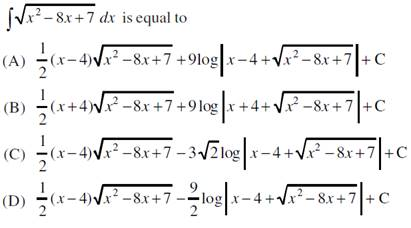Ans.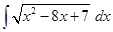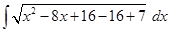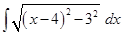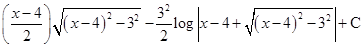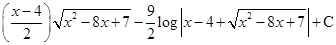hance, option (D) is correct.

Important Study materials for classes 06, 07, 08,09,10, 11 and 12. Like CBSE Notes, Notes for Science, Notes for maths, Notes for Social Science, Notes for Accountancy, Notes for Economics, Notes for political Science, Noes for History, Notes For Bussiness Study, Physical Educations, Sample Papers, Test Papers, Mock Test Papers, Support Materials and Books.Mathematics Class - 11th

NCERT Maths book for CBSE Students.

books

## Study Materials List:

##### Solutions ⇒ Class 12th ⇒ Mathematics-II
7. Integrals
8. Application of Integrals
9. Differential Equations
10. Vector Algebra
11. Three Dimensional Geometry
12. Linear Programming
13. Probability

New Books